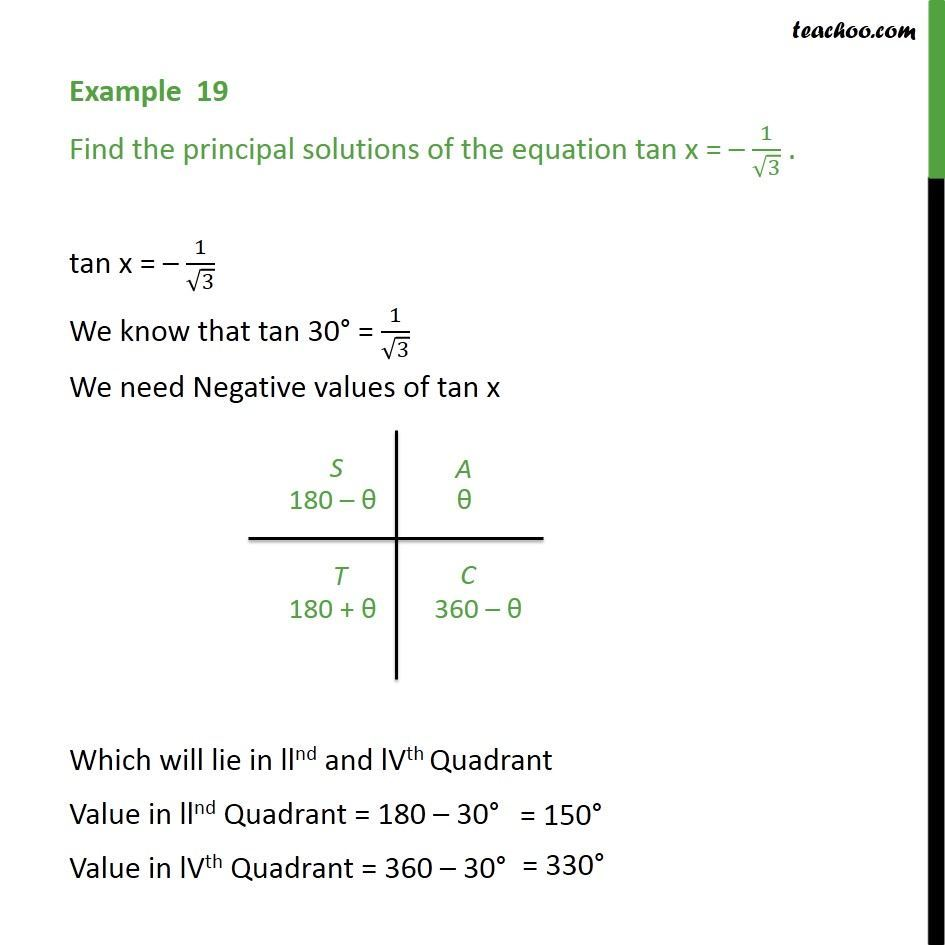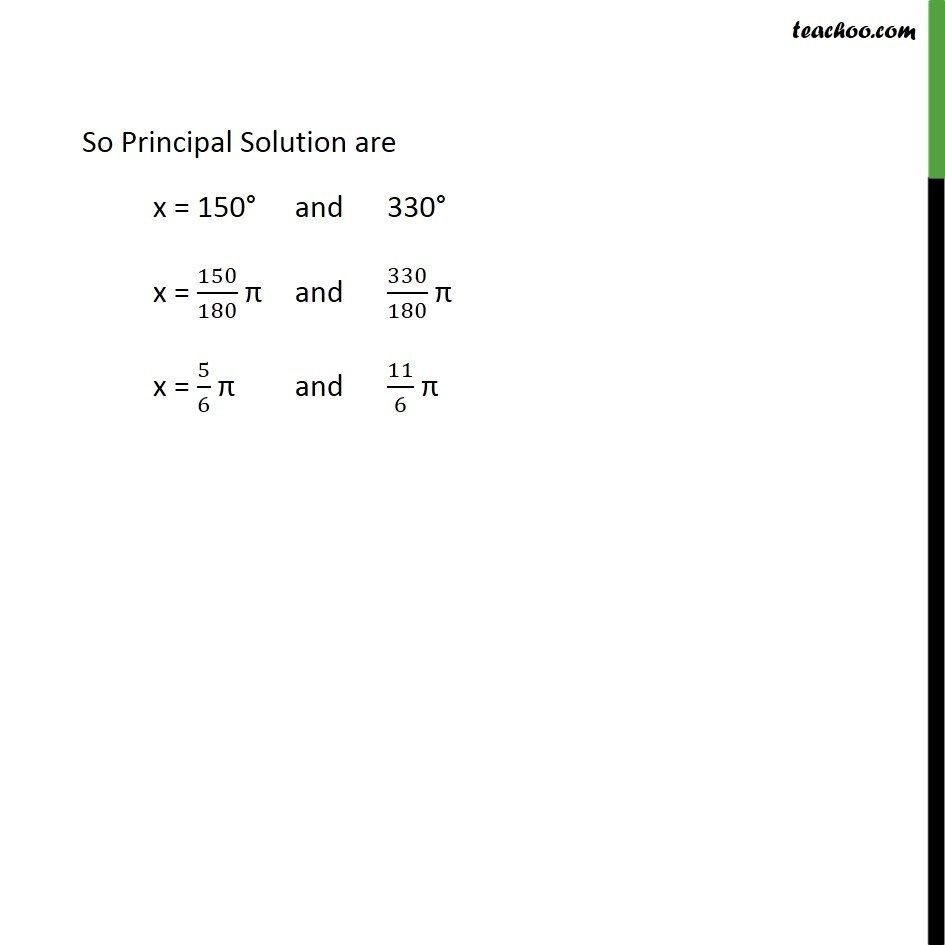1. Chapter 3 Class 11 Trigonometric Functions
2. Serial order wise
3. Examples

Transcript

Example 19 Find the principal solutions of the equation tan x = – 1/√3 . tan x = – 1/√3 We know that tan 30° = 1/√3 We need Negative values of tan x Which will lie in llnd and lVth Quadrant Value in llnd Quadrant = 180 – 30° Value in lVth Quadrant = 360 – 30° So Principal Solution are x = 150° and 330° x = 150/180 π and 330/180 π x = 5/6 π and 11/6 π

Examples